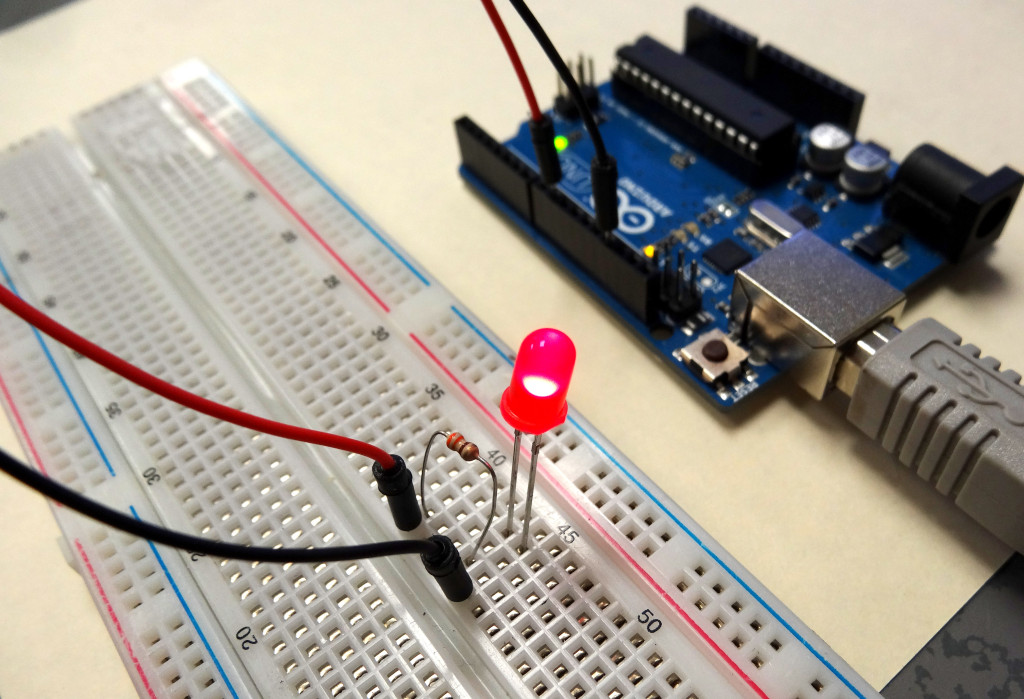# Arduino Tutorial 4: Understanding Arduino Variables

In our earlier lessons we would program using “constants” when we needed numbers. For example, if we wanted to set pin 13 to an output, we would use the command:

pinMode(13, OUTPUT);

The problem with using constants like the number 13, if you decided later to use pin 8 instead, you would have to edit every line of code that used that number. It is much better practice to use variables when coding. In this video we show you have to program using variables. We start by doing things the wrong way, using constants, then show you the advantages of using variables.

Hopefully you can see from this video how much better it is, and more efficient to use variables instead of constants. In all the future lessons, we expect you to use variables.

# Arduino Tutorial 3: Understanding How Breadboards WorkThe cool thing about the arduino is you can program it to interact with the real world. The arduino can connect to and interact with a variety of sensors and actuators which allow you to monitor what is happening around you, and to control things like motors, relays, and servos. To do this though, you need to be able to connect components to the arduino. For prototyping, that is most easily done by using a breadboard. If you want to play along at home, and follow all these lessons with the same components I am using, you can order this Arduino kit.

The video below describes in detail how to use a breadboard, and even helps you get your first circuit prototype up and running.

As explained in the video, key to using the breadboard to create circuits is to understand how the little holes on the breadboard are connected. This graphic will help you understand which holes are connected and which are not.